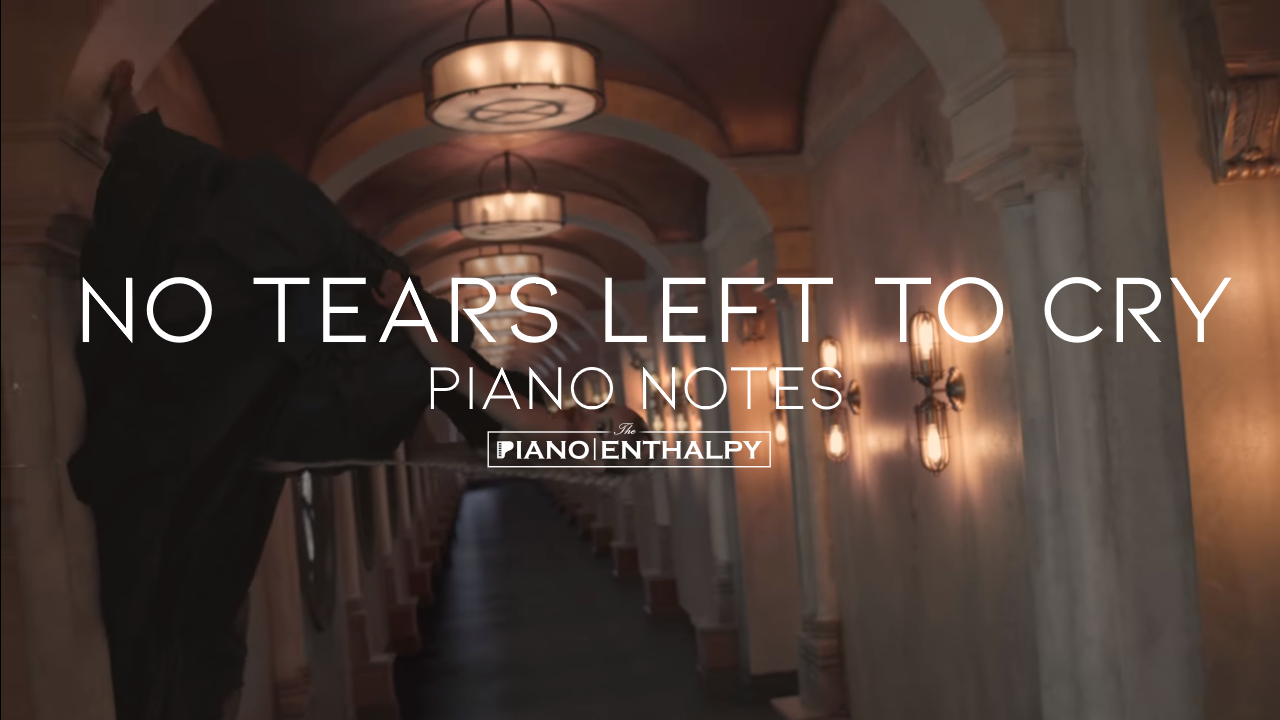# Ariana Grande - No Tears Left To Cry | Piano NotesAriana Grande - No Tears Left To Cry | Piano Notes

Right now, I'm in a state of mind
C++C++, B+B+B+A+B+A+A+ B+C++
I wanna be in like all the time
C++C++C++D++B+ B+A+A+G+E+
Ain't got no tears left to cry
E+E+E+F+G+A+G+ D+E+
So I'm pickin' it up, pickin' it up
C+ C+D+E+C+E+, D+E+C+E+
I'm lovin', I'm livin', I'm pickin' it up
C+D+E+, C+D+E+, C+D+E+C+E+
I'm pickin' it up, pickin' it up
C+D+E+C+E+, D+E+C+E+
I'm lovin', I'm livin', I'm pickin' it up
C+D+E+, C+D+E+, C+D+E+C+E+
I'm pickin' it up, pickin' it up
C+D+E+C+E+, D+E+C+E+
I'm lovin', I'm livin', I'm pickin' it up
C+D+E+, C+D+E+, C+D+E+C+E+
Yeah, we turnin' it up
C+, AAAAA

Ain't got no tears in my body
BBBBBBBBB
I ran out, but boy, I like it, I like it, I like it
ABA, BD+, C+A, AE+A, BC+BA
Don't matter how, what, where, who tries it
BBBBBBBBB
We're out here vibin', we vibin', we vibin'
ABABD+C+A, AE+A, BC+BA

Comin' out, even when it's rainin' down
E+C+D+, G+G+A+G+A+A+GE+
Can't stop now, can't stop so shut your mouth
E+C+D+, B+G+G+E+E+ D+C+A
Shut your mouth, and if you don't know that now
E+C+D+, G+G+A+G+A+G+A+
You know it, babe
B+G+A+
Know it, babe, yeah
E+C+D+A

Right now, I'm in a state of mind
C++C++, B+B+B+A+B+A+A+ B+C++
I wanna be in like all the time
C++C++C++D++B+ B+A+A+G+E+
Ain't got no tears left to cry
E+E+E+F+G+A+G+ D+E+
So I'm pickin' it up, pickin' it up
C+ C+D+E+C+, D+E+C+E+
I'm lovin', I'm livin', I'm pickin' it up
C+D+E+, C+D+E+, C+D+E+C+E+
I'm pickin' it up, pickin' it up
C+D+E+C+, D+E+C+E+
I'm lovin', I'm livin', I'm pickin' it up
C+D+E+, C+D+E+, C+D+E+C+E+